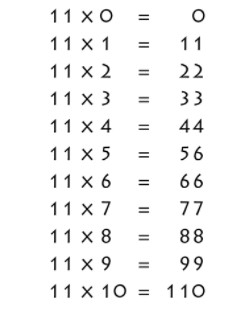# 11 Times Table- Learn Table Of 11 : Multiplication Table Of 11

Safalta expert Published by: Yashaswi More Updated Tue, 01 Mar 2022 09:37 AM IST

## Highlights

Check out how to learn 11 times table easily here at Safalta.com

11 times table is the multiplication table of 11 where we get the product of multiplying 11 with whole numbers. Learning 11 times table is comparatively easier. The multiples are always a repetition of the same digit like, 11 × 1 = 11, 11 × 2 = 22, 11 × 5 = 55, 11 × 79 = 99, etc. In this mini-lesson, you can find the 11 times table, that is, multiplication of 11 with whole numbers up to 10. Join Safalta School Online and prepare for Board Exams under the guidance of our expert faculty. Our online school aims to help students prepare for Board Exams by ensuring that students have conceptual clarity in all the subjects and are able to score their maximum in the exams.

1.

### Free Demo ClassesSource: Safalta.com

11 Times Table Chart
2. Multiplication Table Of 11
3. 11 Times Table
4. Tips for 11 times table
5. Table of 11 upto 20

## 11 Times Table Chart:## Multiplication Table of 11

Multiplication table of 11 is an important skill while solving mathematical problems based on multiplication and division. It is very helpful to find the multiples of 11. Go through the 11 times table that is given below for fast calculations.

## Tips for 11 Times Table

1. The first nine multiples of 11 are very easy to memorize. When 11 is multiplied by any single digit natural number, the product of that natural number is written twice. For example, 11 × a = aa; here a is a natural number. Like this, we have obtained the first nine multiples of 11 and hence, the 11 times table.

2. To calculate the 11 times table for 2 digits numbers, there is a trick. When a two-digit number is multiplied by 11, take the sum of the digits of the number and place the sum between the two digits of the original two-digit number. For example, when 11 is multiplied by 16, take the sum of 1 and 6, that is, 7. Now, place the sum 7 between 1 and 6. The resulting number is 176. The product of 11 and 16 is 176, that is, 11 × 16 = 176.

## Worksheets on 11 Times Table

1. ### Example 1: Using 11 times table, evaluate 11 times 7 minus 2 plus 4.

Solution:

First, we will write 11 times 7 minus 2 plus 4 mathematically.

Using 11 times table, we have: 11 times 7 minus 2 plus 4 = 11 × 7 - 2 + 4= 77 + 2 = 79

Hence, 11 times 7 minus 2 plus 4 is 79.

2. ### Example 2: Mary went to an orphanage and distributed 5 cupcakes each to the kids there. There were 11 kids in the orphanage. Using Table of 11 find how many cupcakes were distributed in total?

Solution:

There were 11 kids, and she distributed 5 cupcakes each to the kids.

Therefore, from the table of 11, 5 × 11= 55 cupcakes

Hence, 55 cupcakes were distributed in total.

## Multiplication Tables

 2 Times Table 12 Times Table (Soon) 3 Times Table 13 Times Table (Soon) 4 Times Table 14 Times Table (Soon) 5 Times Table 15 Times Table (Soon) 6 Times Table 16 Times Table (Soon) 7 Times Table 17 Times Table (Soon) 8 Times Table 18 Times Table (Soon) 9 Times Table 19 Times Table (Soon) 10 Times Table 20 Times Table (Soon)

## What is the importance of learning Table 2 to 20?

For making mathematical section easier, memorising table 2 to 20 is important.

## How can I learn tables easily?

Daily recite the table as mentioned in the article twice and thrice.

## Which is the most easiest table?

11 times table is one of the most easiest tables to learn.

## What is the 11 times table?

Learning 11 times table is comparatively easier. The multiples are always a repetition of the same digit like, 11 × 1 = 11, 11 × 2 = 22, 11 × 5 = 55, 11 × 79 = 99, etc.

## Is 154 in the 11 times table?

The above chart will help us to read and write the 11 times table. Now we will learn how to do forward counting and backward counting by 11's. Forward counting by 11's: 0, 11, 22, 33, 44, 55, 66, 77, 88, 99, 110, 121, 132, 143, 154, 165, 176, 187, 198, 209, 220, 231, 242, 253, 264, 275.